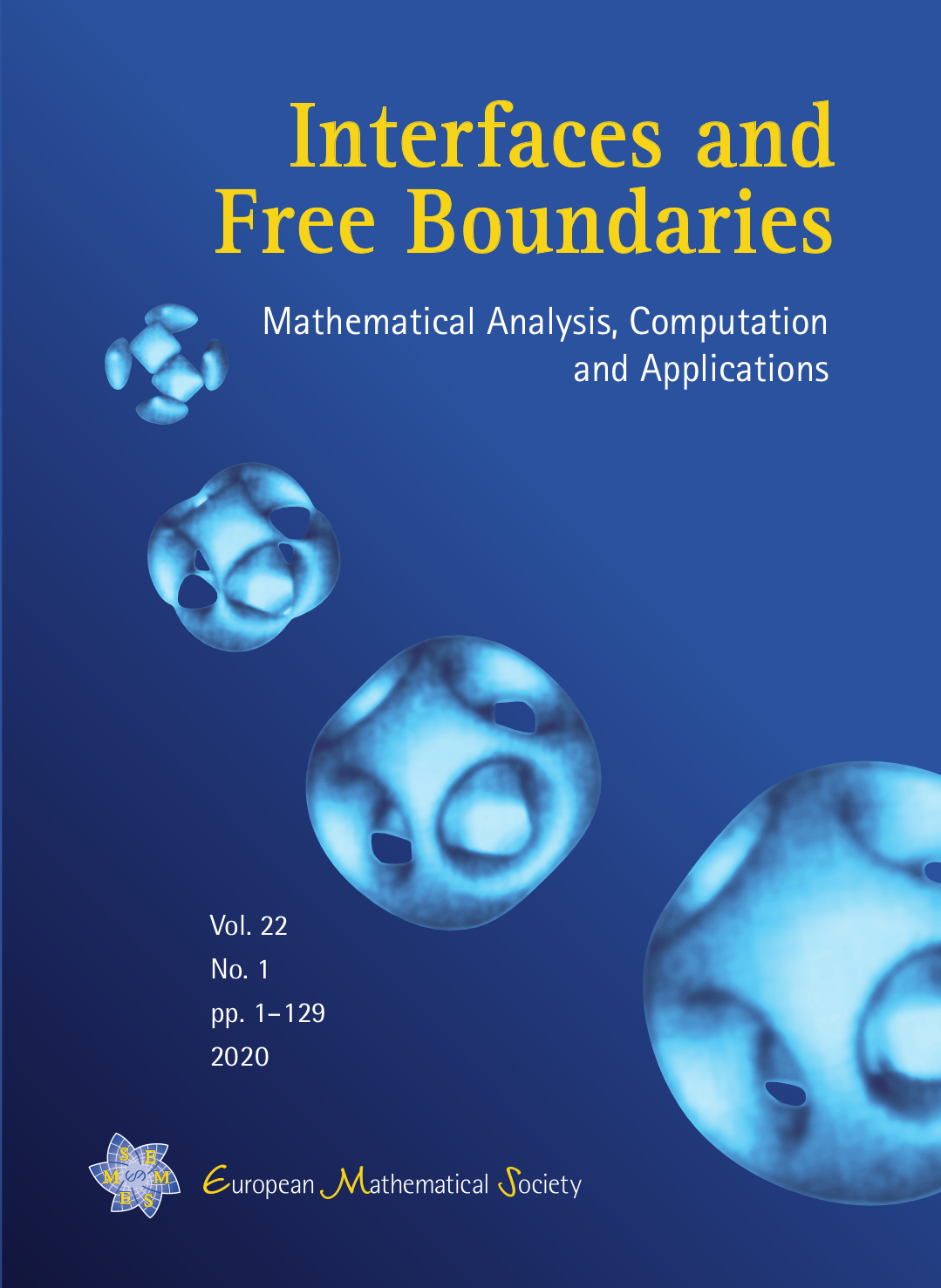IFB

# Interfaces and Free Boundaries

DOI: 10.4171/IFB | ISSN: 1463-9963 | eISSN: 1463-9971Interfaces and Free Boundaries is dedicated to the mathematical modelling, analysis and computation of interfaces and free boundary problems in all areas where such phenomena are pertinent. The journal aims to be a forum where mathematical analysis, partial differential equations, modelling, scientific computing and the various applications which involve mathematical modelling meet. Submissions should, ideally, emphasize the combination of theory and application.

Interfaces and Free Boundaries is covered in:
Mathematical Reviews (MR), Current Mathematical Publications (CMP), MathSciNet, Zentralblatt für Mathematik, Zentralblatt MATH Database, Science Citation Index Expanded (SCIE), CompuMath Citation Index (CMCI), Current Contents/Physical, Chemical & Earth Sciences (CC/PC&ES), ISI Alerting Services, Journal Citation Reports/Science Edition, Web of Science.

This journal adheres to the EMS Code of Practice.